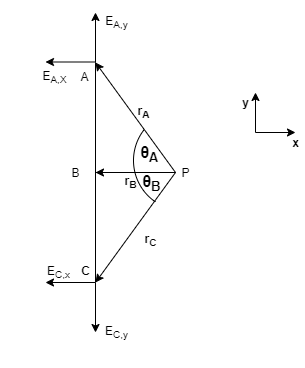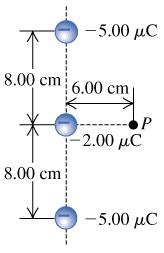# Problem: Three negative point charges lie along a line as shown in the figure. Find the magnitude of the electric field this combination of charges produces at point P, which lies 6.00 cm from the -2.00 μC charge measured perpendicular to the line connecting the three charges. (E = N/C)Find the direction of the electric field this combination of charges produces at point P, which lies 6.00 cm from the -2.00 μC charge measured perpendicular to the line connecting the three charges. outward or inward -2.00 μC?

###### FREE Expert Solution

Electric field due to a point charge:

$\overline{){\mathbf{E}}{\mathbf{=}}\frac{\mathbf{k}\mathbf{q}}{{\mathbf{r}}^{\mathbf{2}}}}$rA = rC = (62 + 82)(1/2) = 10 cm(1m/100cm) = 0.1 m

rB = 6 cm(1m/100cm) = 0.06 m

1 μC = 10-6 C

θA = θB = tan-1 (y/x) = tan-1 (8cm/6cm) = 53.1°

91% (28 ratings)###### Problem Details

Three negative point charges lie along a line as shown in the figure.Find the magnitude of the electric field this combination of charges produces at point P, which lies 6.00 cm from the -2.00 μC charge measured perpendicular to the line connecting the three charges. (E = N/C)

Find the direction of the electric field this combination of charges produces at point P, which lies 6.00 cm from the -2.00 μC charge measured perpendicular to the line connecting the three charges. outward or inward -2.00 μC?